## Math Fact Resources

### What are Math Facts?

Math facts are defined as addition of single digit numbers (1+2, 4+3, 7+9 etc..), subtraction of corresponding addition sums (18-7, 2-1, 12-3), multiplication up to 10, and corresponding division of those products. Math fact fluency is considered a building block to higher level math. Efficient and accurate recall of math facts builds confidence and frees up working memory for more complex math problem solving. The goal of math fact fluency is not teaching students to just memorize math facts but to build number-sense based strategies to determine math facts and with practice – to help build efficiency, flexibility and accuracy with math facts.

Addition Strategies include: Count on from the higher number - for example, when adding 1+7, count on from the higher number, 7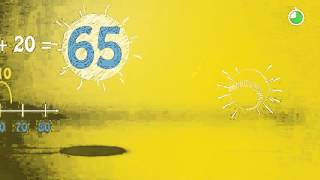Bridge to Ten - When adding two numbers together where one number is close to 10, count on to 10 and add what is remaining of the 2nd addend to 10. 9+5= 9+1+4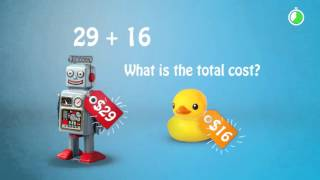Doubles Plus One - When adding two addends one digit apart, double the lesser addend and add one 7+8 = 7+7+1 Doubles Plus Two - When adding two addends two digits apart, double the lesser addend and add two 7+9 = 7+7+2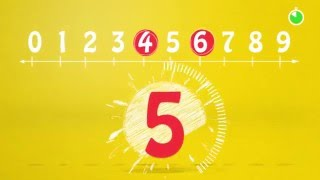### Subtraction Strategies

Use the unknown addend model for subtraction - if your equation is 12-9 use what you know about addition to solve "what plus 9 = 12? It's 3!"This can be expanded into much greater number equations like 63-44 = Build up from 44 to get to 63 to solve 44+6=50 and 50+13=63 so the difference is 13+6=19

1. Rising 1st Grader – Identify numbers 0-30 and build number combinations 0-10 using manipulatives
2. Rising 2nd Grader – Automatic recall of addition facts up to 9+9
3. Rising 3rd Grader – Automatic recall of addition & subtraction facts up to 20
4. Rising 4th Grader – Automatic recall of multiplication fact families through 10 and corresponding division facts
5. Rising 5th Grader – Automatic recall of all multiplication facts up to 12 and corresponding division facts

### Multiplication Strategies

Double, Double, Double - use what you already know to find the product x2 - Take the factor other than 2 and double it 8x2 is 8 doubled which is 16 x4 - Take the factor other than 4 and double, double 8x4 = 8 doubled which is 16 and 16 doubled which is 32 x8 - Take the factor other than 8 and double, double, double 8x8 = 8 doubled which is 16, 16 doubled which is 32 and 32 doubled which is 64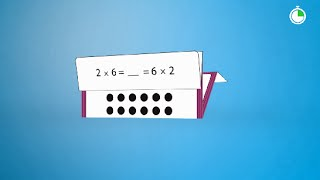x5 - Take what we know about x10 and half it to know our x5 facts 8x5 = we know 8x10 is 80 and if we have 1/2 that many 8s (5 instead of 10) it must be 1/2 of 80 which is 40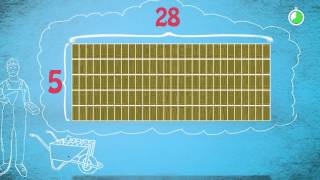You can also use the "clock face" strategy (resources in the side bar) - use what you know about telling time to determine 5 facts - you know that when the 2nd hand is in the 4 that it is 20 past the hour, and you know the 4 represents 4 groups of 5 minutes which is 20 x9 and x6 - Use build up and build down to determine new math facts - If you have 8x6 you can take what you know about 8x5 which is 40 and add another group of 8 so it's 48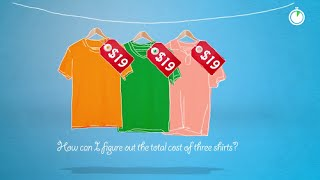### Division Strategies

Use what we know about fact families to help remember division facts. Use what you know about multiplication to solve division facts. 45/9 is 5 because I know that 9 groups of 5 is 45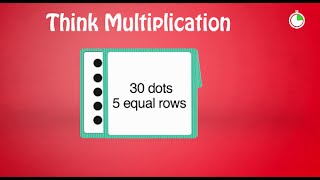You can also use what you already know about your x2, x4 and x8 facts for division facts with those divisors. For example, if 2x6 is 6 doubled (12), then 12/2 must be12 halved which is 6. To extend this strategy, if you are dividing by 8, take the dividend and half, half, half it - for example 64/8 = 64 halved is 32, 32 halved is 16 and 16 halved is 8.

Ten Frame Mat

Number Line 1-20

Double, Double, Double Mat

Clock Face Game

DIY Math Game Ideas

Math Vocabulary Sheet